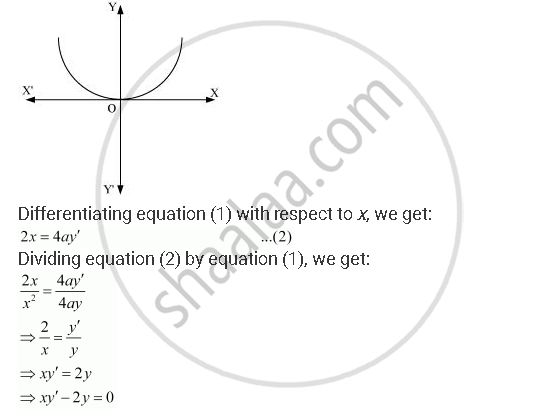Share

# Form the Differential Equation of the Family of Parabolas Having Vertex at Origin and Axis Along Positive Y-axis. - CBSE (Commerce) Class 12 - Mathematics

ConceptProcedure to Form a Differential Equation that Will Represent a Given Family of Curves

#### Question

Form the differential equation of the family of parabolas having vertex at origin and axis along positive y-axis.

#### Solution

The equation of the parabola having the vertex at origin and the axis along the positive y-axis is:

x^2 = 4ay  ......(1)This is the required differential equation.

Is there an error in this question or solution?

#### APPEARS IN

NCERT Solution for Mathematics Textbook for Class 12 (2018 to Current)
Chapter 9: Differential Equations
Q: 7 | Page no. 391
Solution Form the Differential Equation of the Family of Parabolas Having Vertex at Origin and Axis Along Positive Y-axis. Concept: Procedure to Form a Differential Equation that Will Represent a Given Family of Curves.
S